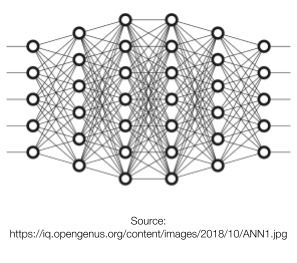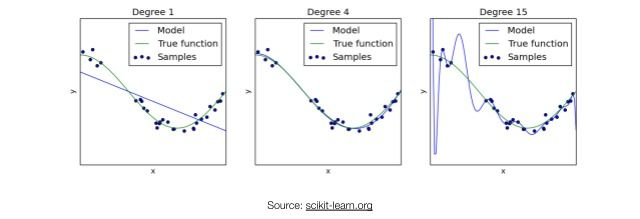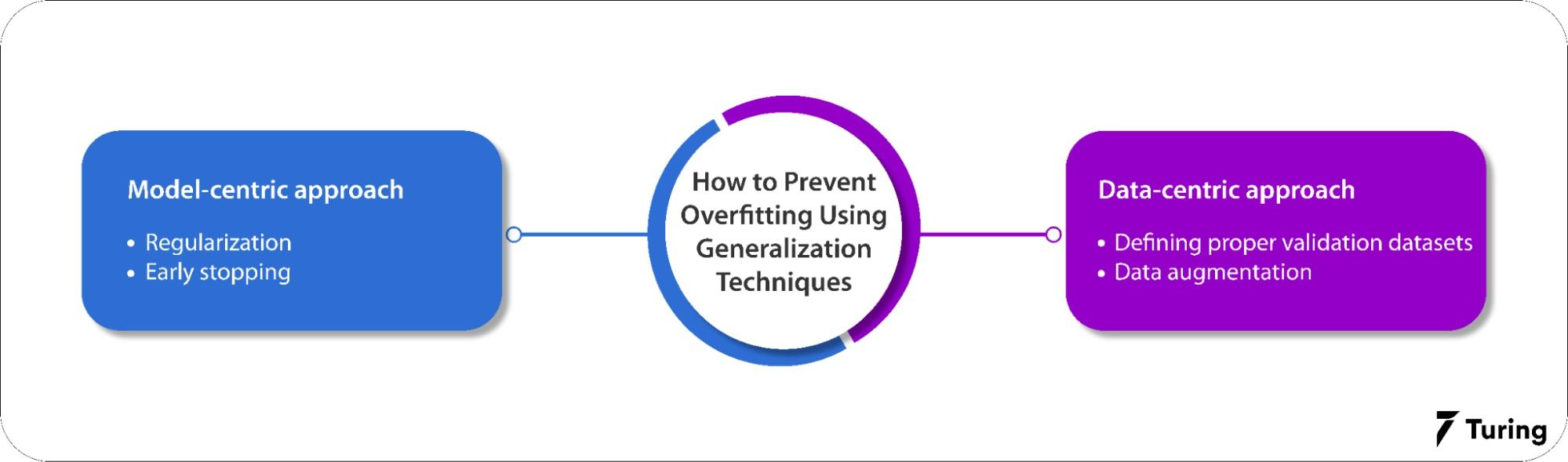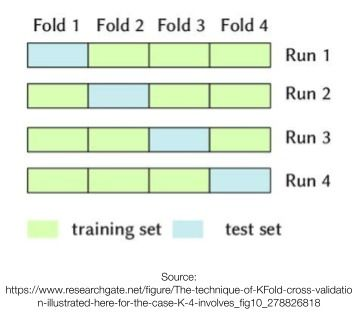# Complete Guide to Making Deep Learning Models Generalize BetterDeep learning models have the power to analyze and understand patterns present in data. But they are also susceptible to overfitting as the patterns learnt are only as good as the data they are trained on. Hence, there is a need for techniques to prevent overfitting. These methods are called generalization. In this blog, we will explore the different methods used to train deep learning models to help them generalize better on unseen data. Before that, however, we will look at how deep learning models are trained and what aspects we can improve during training to enhance generalization.

## What are deep learning models?

Deep learning models can be understood as a black box that tries to figure out the pattern/relationship between dependent variables (targets) and independent variables (features). These models consist of multiple layers of neurons or weights that are updated according to the inputs and targets using a technique called gradient descent.

## How do neural networks work?

A neural network is a network of mathematical equations that consists of multiple neurons interconnected with each other through various layers to form a deep neural architecture.

Following is an example of the architecture of a deep neural network:Image source: OpenGenus IQ

The above image depicts multiple neurons/nodes with different weights interconnected to form a dense neural network. The deep learning model is fed with the training data at the input layers which propagates through the network via hidden layers and finally generates the output at the output layers. The loss is calculated from the generated output. Depending on the loss, the weights are updated. This process is called backpropagation and this is where the model learns the patterns from the data.

Even though the deep learning model is trained on the training data, we won’t have a clear idea of how well it will perform on the unseen data, i.e., the data that is not used to train the model. At this point, we have to introduce some techniques - generalization - so that it performs well on the unseen data.

## What is generalization and why is it needed?

Generalization is the ability of a deep learning model to learn and properly predict the pattern of unseen data or the new data drawn from the same distribution as that of the training data. In simpler words, generalization defines how well a model can analyze and make correct predictions on new data after getting trained on a training dataset.

Let’s explore the variance and bias of a model and see how it affects the generalization capability.

Variance and bias are two crucial terms in machine learning. Variance defines the variability of predictions made by the model, i.e., how far a set of numbers are spread out from their actual value. Bias defines the distance of the predictions from their actual values.

Every machine learning model usually comes under any one of the following stages:

• Low bias - Low variance
• Low bias - high variance
• High bias - Llow variance
• High bias - high variance

In the above stages, the low bias-high variance model is called the overfitted model and the high biase-low variance model is called the underfitted model. Underfitting and overfitting can be explained in the graph below:Image source: scikit-learn

In the figure above, the first graph represents the underfitted model, i.e., the model has not learned the patterns of the training data and cannot generalize properly on new data. The second figure represents the correct-fit model. This means it has properly identified the patterns of training data. The third figure represents the overfitted model. Here, the model has learned the exact patterns of the training data such that it fails to generalize on unseen data.

Through generalization, we can find the best trade-off between underfitting and overfitting so that a trained model performs to expectations.

## Generalization techniques to prevent overfitting in deep learning

In this section, we will explore different generalization techniques to ensure that there is no overfitting in the deep learning model. Various approaches can be categorized under data-centric and model-centric generalization techniques. They ensure that the model is trained to generalize the validation dataset and find required patterns from the training data.### Data-centric approach

The data-centric approach primarily deals with data cleaning, data augmentation, feature engineering and, finally, preparing proper validation and testing datasets.

We will now take a look at some of the most important data-centric generalization techniques: preparing proper validation sets and data augmentation.

#### Defining proper validation datasets

Defining a proper validation dataset is the first step in predictive modeling. This is very important because having a perfect validation set means we will have a really good representation of real-world data. It will be easy to evaluate our machine learning model and detect whether it is generalizing or not.

Ideally, the dataset used to train the machine learning model should have a diverse set of data samples which will result in the model learning or detecting as many patterns as possible from the data. The performance of the model also depends on the number of data samples available. Usually, deep learning models in computer vision and natural language processing (NLP) applications are trained on millions and millions of data samples (images or text) to ensure higher model generalization.

In addition, during training, it is recommended that cross-validation techniques like K-fold or stratified K-fold are used to enable better learning on the training dataset. Cross-validation techniques yield brilliant results because they enable the model to learn from the entire dataset while simultaneously using it for both training and validation.

Below is an example of K-fold cross-validation technique:Image source: ResearchGate

#### Data augmentation

Data augmentation is a technique that is generally used to improve a model’s performance. It comprises a set of methods used to artificially increase the number of data samples present in the dataset. This is done because deep learning models generalize well when the number of data samples available to train on is more. In this way, we can create state-of-the-art models with fewer data samples available.

The data augmentation technique is applied to computer vision applications where domain-specific data, such as medical data, is not abundantly available.

### Model-centric approach

The model-centric approach defines various methods that can be used to improve the performance of machine learning models during training and inference. Some of the techniques are:

#### Regularization

This is one of the most important generalization techniques. Regularization is used to address overfitting by directly changing the architecture of the model, thereby modifying the training process. There are three types of regularization techniques: L1, L2, and dropout regularization. They ensure that the model is not overfitted by modifying the way the parameters or weights are updated.

#### Early stopping

Early stopping is a technique used to prevent the model from overfitting during training. Generally, the model learns from the training dataset by optimizing a loss function through gradient descent. This happens in an iterative manner, i.e., the model is trained for a number of epochs before it converges. Early stopping is used to prevent overfitting by stopping the model training when the validation loss increases over a certain defined point.

We have seen how deep learning models are trained and the techniques used to make them generalize better. Employing these techniques when training a model significantly increases its performance and generalization capability. This capability is important because it defines how much the model can be applied in the real world. Therefore, it is always recommended that the techniques discussed above are used to properly train a model before deployment

## Author

•### Turing

Author is a seasoned writer with a reputation for crafting highly engaging, well-researched, and useful content that is widely read by many of today's skilled programmers and developers.

Generalization of deep learning models can be improved by defining proper validation datasets and implementing data augmentation, regularization, and early stopping in the deep learning/machine learning model training loop.

Here are some tips to make a deep learning model more accurate:

• Collect abundant data.
• Include more layers to the model.
• Choose a common image size.
• Increase epochs.
• Reduce color channels.

Overfitting in deep learning models can be prevented by simplifying the data, using data augmentation, dropouts, regularization, early stopping, and other techniques.### Press

What's up with Turing? Get the latest news about us here.### Blog